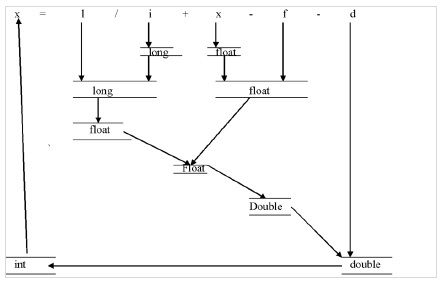# What are implicit and explicit type conversions in C language?

Converting one data type into another data type is called type conversions.

• Implicit type conversion
• Explicit type conversion

## Implicit type conversion

• The compiler provides implicit type conversions when operands are of different data types.

• It is automatically done by the compiler by converting smaller data type into a larger data type.

int i,x;
float f;
double d;
long int l;Here, the above expression finally evaluates to a ‘double’ value.

## Example

Following is an example for implicit type conversion −

int x;
for(x=97; x<=122; x++){
printf("%c", x); /*Implicit casting from int to char %c*/
}

## Explicit type conversion

• Explicit type conversion is done by the user by using (type) operator.

• Before the conversion is performed, a runtime check is done to see if the destination type can hold the source value.

int a,c;
float b;
c = (int) a + b

Here, the resultant of ‘a+b’ is converted into ‘int’ explicitly and then assigned to ‘c’.

## Example

Following is an example for explicit type conversion −

int x;
for(x=97; x<=122; x++){
printf("%c", (char)x); /*Explicit casting from int to char*/
}

Let us see the difference between the two types of conversions with examples −

## Example (Implicit conversion)

Live Demo

#include<stdio.h>
main(){
int i=40;
float a;
//Implicit conversion
a=i;
printf("implicit value:%f",a);
}

## Output

Implicit value:40.000000

## Example (Explicit Conversion)

Live Demo

#include<stdio.h>
main(){
int i=40;
short a;
//Explicit conversion
a=(short)i;
printf("explicit value:%d",a);
}

## Output

Explicit value:40